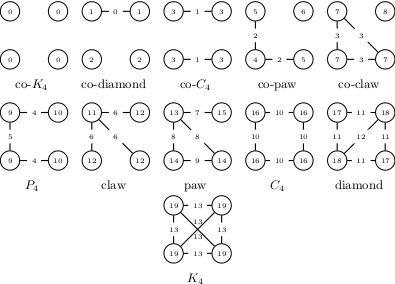## Contents

### MethodAll non-isomorphic subgraphs with four nodes (quads). Node and edge labels refer to the orbits and were enumerated such that each orbit is identified with a single quad.

The frequency distribution over all non-isomorphic 4-node subgraphs is called the quad census.

The quad census analysis calculates for each node and edge respectively how often it is contained in a specific quad. Depending on the chosen parameters this analysis further distinguishes between different orbits. For example a claw has two node orbits, i.e., 11 and 12. Considering the orbits leads to a finer grained description of the nodes/edges and allows for a more precise comparison.

### Complexity

The computation of the orbit-aware Quad Census can be done inwheredenotes the number of edges of the graph andis the arboricity of the graph.

More detailed information on the computation of the orbit-aware Quad Census is available in

### Settings

• orbit-aware frequencies: If checked the node and edge orbit frequencies are computed, otherwise orbits will not be distinguished.
• write non-induced frequencies: If checked besides the induced also the node and edge (orbit-aware) non-induced frequencies are written.
• write network frequencies: Additionally computes the quad census on a graph level (if checked)

### Result

The result of this analysis are written to node/edge and network attributes:

• ind_orbit_..: The induced orbit-aware frequencies. The orbit number corresponds to the numbering shown in the opposing figure
• non-ind_orbit_...: Same as ind_orbit_.. but for non-induced frequencies
• <quad_name>: If the orbit-aware frequencies box is not checked the names of the corresponding quads is used, e.g. claw or diamond